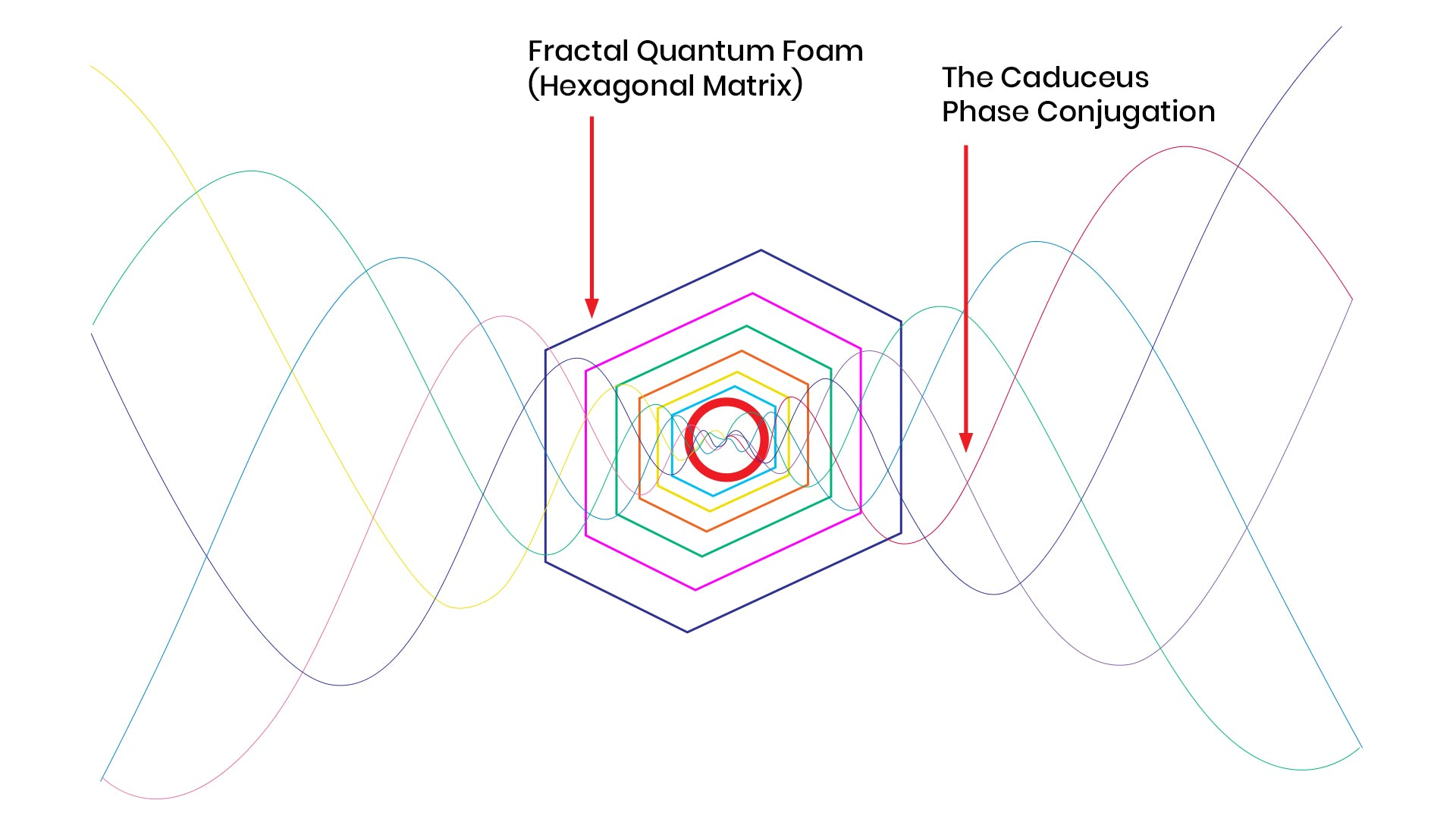Qi Coil™ Matrix (1 Stand) – QiEnergyTech.com

# Qi Coil™ Matrix (1 Stand)

Prix normal
\$199.00
Prix réduit
\$199.00
Prix normal
\$299.00
Épuisé
Prix unitaire
par## What is Phase Conjugation?

Phase conjugation is originally and classically understood in the optics literature. You take pairs of laser beams precisely approaching in opposite directions. If they meet (and conjugate') perfectly - then at the center - they Phase Conjugate. So how do lasers meet to conjugate? When they meet in precise opposing pairs - this is often called four-way mixing. Getting opposing exactly phase-locked laser beams to align this way, is very tricky, and very expensive because you need alignment accuracy down to angstrom levels. We have often suggested that the best way to visualize how wavefronts meet to phase conjugate is to visualize pine cones learning to kiss noses.## The "Holy Grail" of Fusion / ImplosionAs the wave proceeds inward on the cone- in order to create only constructive wave interference, the sine waves must converge the golden ratio proportions. The point here is that at each cross point of the waves- the wave nodes- the wave interference phenomenon both adds and multiplies. You can see visually how the golden proportion invites this recursive constructive wave interference to proceed theoretically to some "infinite" limit. Actually our equations for the wavelengths and frequencies of all self-organizing phenomenon, especially life force (in the form of photosynthesis dimensions- etc.), strongly suggest that this "infinite" limit is definitely the Planck time and length dimension. This makes sense as physics rather agrees the Planck wave dimensions in time and space are the common musical key signature of all of matter (literally the dimensions of the "sacred").

What is especially important to point out here is that golden ratio constructive wave interference in this geometry is not just constructively adding and multiplying wave length, but in fact and perhaps more importantly, is a significant component of the charge wave inertia ( in golden ratio hydrogen for example) know as phase velocity. So a portion of the wave that was undergoing constructive compression by golden ratio is now also undergoing acceleration. In this way - the golden ratio - allows a portion of the constructive compression of charge to turn into the constructive acceleration of that charge. Although some would quibble I still believe that in fact the acceleration of charge is in truth indistinguishable from gravity. In other words this is the origin of acceleration of charge which we call gravity.

## The Phase ConjugationBy having Qi coilspositioned on the Qi Coil Matrix, and with opposite spin density directions, it produces a conjugate field that cascades into a focal point by utilizing nature golden mean ratio (Phi), allowing for the adding, multiply, steering, and collapse (centripetal) of our frequency-modulated electromagnetic healing waveforms.

The resultant longitudinal/compressional EMF is demonstrably superluminal / faster than light: this enables remote action at a distance. This "way out" (thru lightspeed) for a charge during perfect non-destructive conjugate implosive charge compression - is the mechanism/cause of gravity, electronegativity, life force, consciousness, ALL negentropy.

## The Centripetal Charge CompressionIt originates scaled precisely to Planck Length & Planck Time multiplied precisely by powers of Golden Ratio. This Phase Conjugation adds & multiplies constructively phase Velocities converting Compression in Acceleration, the gravity. It is also the proven geometry of the DNA, Earth's magnetic lines, and arrangement of masses in the UNIVERSE!

Biological systems use common electromagnetism, frequency modulation, amplitude modulation, and also use this "time-domain" type of "hidden" electrodynamics. In fact, it uses it to heal damaged cells, etc. When these strange kinds of electromagnetic waves would suddenly appear in experiments (1972) and "restore order", modern phase conjugate optics was born.

## INCLUDES

• 1 Matrix stand
*images with Qi Coil and Powerkit is for representation only, Qi Coil and Powerkit SOLD SEPARATELY

## Works with all Qi Coils

• 6 Qi Coil Max (3 yin/ 3yin) with 6 stands with amp
• Qi Coil 3s (3 yin/ 3yin) with 6 stands with amp
• 6 Qi Coil 2s (3 yin/ 3yin)  with 6 stands with amp

Back to the top